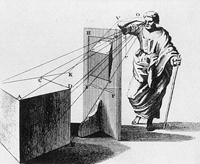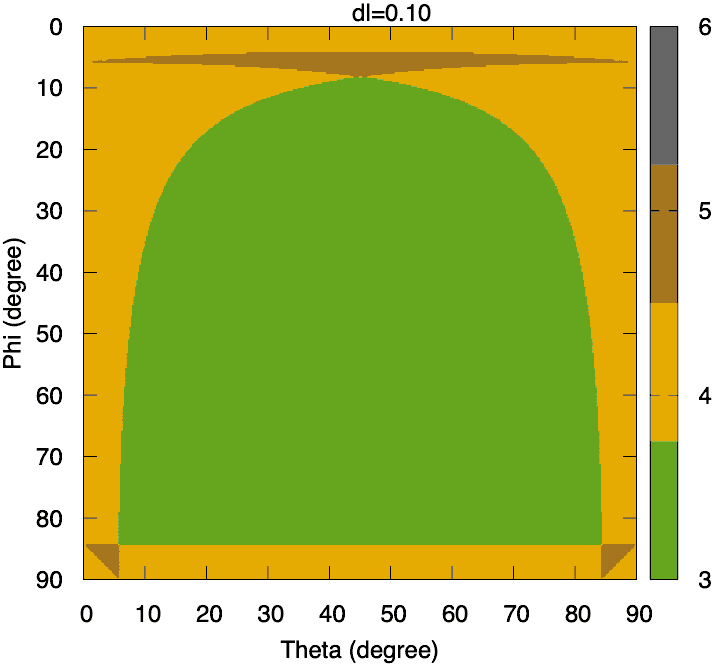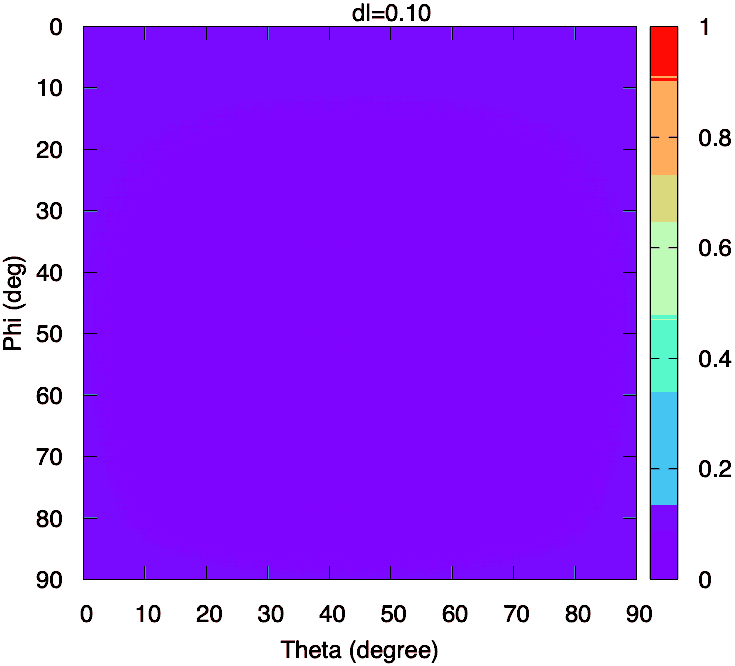Cococubed.com Volume from a plane slicing a cube

Home

Astronomy research
Software instruments
Stellar equation of states
EOS with ionization
EOS for supernovae
Chemical potentials
Stellar atmospheres

Voigt Function
Jeans escape
Polytropic stars
Cold white dwarfs

Cold neutron stars
Stellar opacities
Neutrino energy loss rates
Ephemeris routines
Fermi-Dirac functions

Polyhedra volume
Plane - cube intersection
Coating an ellipsoid

Nuclear reaction networks
Nuclear statistical equilibrium
Laminar deflagrations
CJ detonations
ZND detonations

Fitting to conic sections
Unusual linear algebra
Derivatives on uneven grids

Supernova light curves
Exact Riemann solutions
1D PPM hydrodynamics
Hydrodynamic test cases
Galactic chemical evolution

Universal two-body problem
Circular and elliptical 3 body
The pendulum
Phyllotaxis

MESA
MESA-Web
FLASH

Zingale's software
Brown's dStar
GR1D code
Herwig's NuGRID
Meyer's NetNuc
Presentations
Illustrations
Public Outreach
Education materials
2023 ASU Solar Systems Astronomy
2023 ASU Energy in Everyday Life

AAS Journals
2023 AAS Peer Review Workshops
2023 MESA VI
2023 MESA Marketplace
2023 MESA Classroom
2023 Neutrino Emission from Stars
2023 White Dwarfs & 12C(α,γ)16O
2022 Earendel, A Highly Magnified Star
2022 Black Hole Mass Spectrum
2022 MESA in Don't Look Up
2021 Bill Paxton, Tinsley Prize

Contact: F.X.Timmes
my one page vitae,
full vitae,
research statement, and
teaching statement.

The intersection of a plane and a cube is a geometric computation with applications in computer graphics, solid modeling, and computational astrophysics (e.g., fraction of cell that may be partial ionized or covered by a burning front).

The tool cube_and_plane.tbz computes the volume of a plane slicing through a cube. This slicing results in an inscribed irregular polygon with 3 to 6 intersection points, as shown below. This tool extends the idea in Salama & Kolb 2005 by constructing an ordered list of the face vertices, and hence the volume of the irregular polyhedron.

On the unit cube with vertices and faces enumerated and ordered as shown by the figure above, there are only three independent paths from the front vertex ${\bf V}_1$ to the back vertex ${\bf V}_8$ as marked by the black, dark gray, and light gray lines. The intersecting plane is defined by the length of the normal ray dl (aka the radius vector), and the spherical angles $0 \le \theta \le 2\pi$ and $0 \le \phi \le \pi$. The Cartesian coordinates of the normal ray are \begin{equation} {\bf n}= [dl \cos(\theta), \ dl \sin(\theta)\sin(\phi), \ dl \cos(\phi) ] \ . \label{eq1} \tag{1} \end{equation} Take the equation of the plane, $ax + by + cd + d = 0)$, to be \begin{equation} dl = {\bf \hat{n}} \cdot {\bf x} \label{eq2} \tag{2} \end{equation} where ${\bf \hat{n}}$ is the unit normal vector. Edge $E_{i \rightarrow j}$ between vertices ${\bf V}_i$ and ${\bf V}_j$ is described by the straight line \begin{equation} E_{i \rightarrow j} = {\bf V}_i + \lambda ( {\bf V}_j - {\bf V}_i ) \label{eq3} \tag{3} \end{equation} and thus the intersection point between the plane and the straight line spanned by $E_{i \rightarrow j}$ is \begin{equation} \lambda = \frac{ dl - {\bf \hat{n}} \cdot {\bf V}_i }{{\bf \hat{n}} \cdot ({\bf V}_j - {\bf V}_i ) } \label{eq4} \tag{4} \end{equation} There is an intersection only if $0 \le \lambda \le 1$, otherwise the plane does not intersect the edge within the cube.

The gif animation on the left shows the number of vertices of the intersecting polygon in the $\theta - \phi$ plane as a function of the length of the normal ray $dl$ and the gif animation on the right shows the volume of a unit cube. Whil the changes in the volume are smooth. the number of vertices of the intersecting polygon show wild patterns.Number of vertices of the intersecting polygon.Volume of the unit cube.

Please cite the relevant references if you publish a piece of work that use these codes, pieces of these codes, or modified versions of them. Offer co-authorship as appropriate.